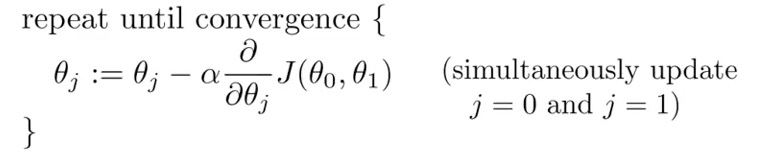# PuGong

## Intro and Linear Regression | Machine Learning

29 Jan 2016

### Intro and Linear Regression

#### Reference:

Course Home in Coursera

Course Homepage in Stanford

Course Materials

Rachel的笔记(中文)

#### Introduction:

The defination of Machine Learning:

• older: the field of study that gives computers the ability to learn without being explicitly programmed

• Modern: A computer program is said to learn from experience E with respect to some class of tasks T and performance measure P, if its performance at tasks in T, as measured by P, improves with experience E

Example: playing checkers.

E = the experience of playing many games of checkers

T = the task of playing checkers.

P = the probability that the program will win the next game.

Tool to used in this course: Matlab / Octave

Supervised Learning: Dataset with labels, know what our correct output should look like, having the idea that there is a relationship between the input and the output.

Regression problem: to predict results within a continuous output, meaning that we are trying to map input variables to some continuous function. Namely the price.

Classification problem: o predict results in a discrete output. In other words, to map input variables into discrete categories, like 0 and 1, true and fasle, 1/2/3/4/etc.

Unsupervised Learning: dataset without lables

#### Linear Regression with One Variable

Cost function: also called square error function, the function to return a number representing how well the neural network performed to map training examples to correct output.Gradient Descent: is a very popular learning mechanism that is based on a greedy, hill-climbing approachalpha: learning rate

Batch Gradient Descent: each step of gradient descent use all the training examples.Convex function： （Bowl-shaped function）#### Linear Regression with Multiple Variables

mean normalization: keep the x -1 ≤ x ≤ 1, just the formula to narrow the values:

``````x¡ = (x - mean of x) / (range of x, maxium of x - minium of x)
``````

feature and ploynomial regression: to choose the suitable features

Feature Scaling: is a method used to standardize the range of independent variables or features of data. In data processing, it is also known as data normalization and is generally performed during the data preprocessing step.

Learning Rate: if learning rate alpha is too small, slow convergence if too large, J(ø) may not decrease on every interation; may not converge.

```q&A:
avg distance    range       value
89      81  8   25      0.32
72      81  -9  25      -0.36
94      81  13  25      0.52
69      81  -12 25      -0.48

avg distance    range       value
7921        6675.5  1245.5  4075        0.305644172
5184        6675.5  -1491.5 4075        -0.36601227
8836        6675.5  2160.5  4075        0.530184049
4761        6675.5  -1914.5 4075        -0.469815951
```

``````need to choose alpha
need many iterations
work well when n is large
``````

Normal Equation:

``````don't need to chosose alpha
don't need to to iterate
need to computer (n*n)
slow if n is very large
``````

#### Octave Tutorial

Octave homepage

Octave Installation Guide

Octave Document:

Octave Reference

Octave Wiki